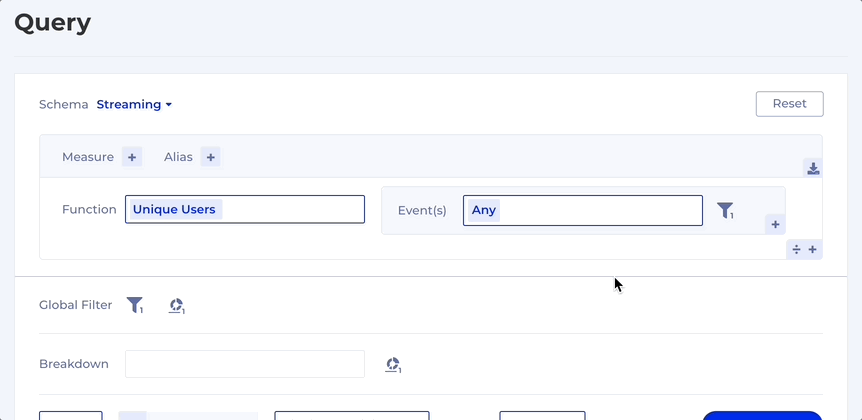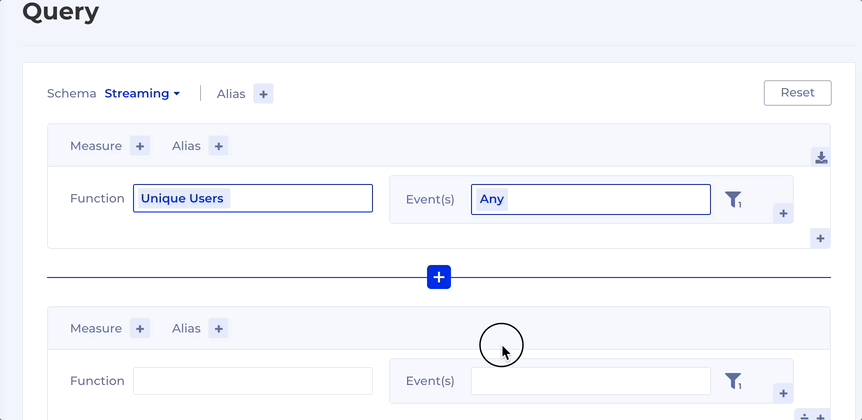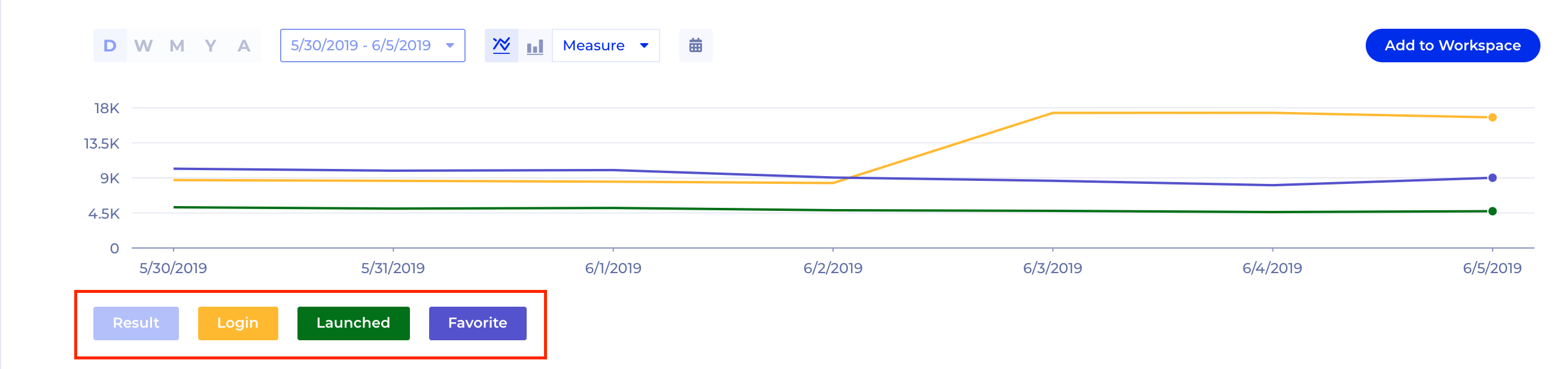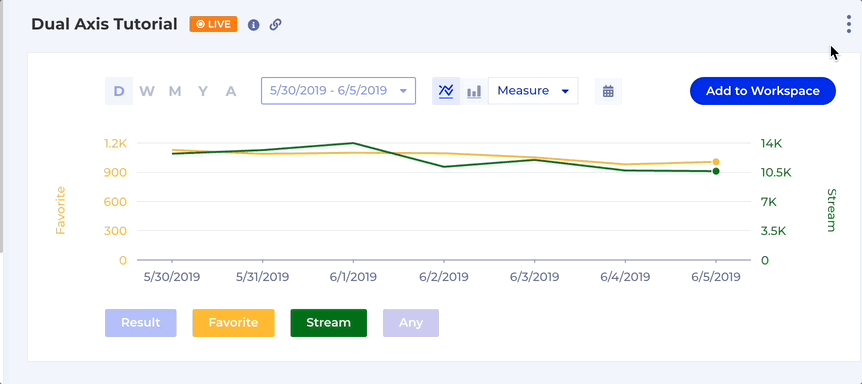# Compound Measures

## Introduction

A Compound Measure is a type of Measure that involve two or more other Simple Measures. For example, customer conversion rate, calculated as a numerator divided by a denominator, is a Compound Measure. Kubit supports Compound Measures using the addition (`+`) and division (`÷`) operators.

## Order of Operations

In any mathematical expression, it is essential to understand the order of operations to get the desired result. In Kubit, there are two operators available, addition (`+`) and division (`÷`). Whenever a division operator is present, Kubit will first evaluate the numerator followed by the denominator and then perform the division operation.

Here are a few examples to illustrate how Kubit calculates a Compound Measure:

• (4 + 6) ÷ (2) = 5
• (4 + 6) ÷ (2 + 3) = 2
• (5) ÷ (4 + 1) = 1
• 5 + 1 + 2 = 8

In mathematical terms, Kubit evaluates the numerator and denominator separately and then performs the division operation.

You can add a Simple Measures to your compound measure by selecting `Add Denominator` or `Add Measure.`## Remove a Simple Measure

You can delete a Simple Measure by hovering over the Measure and selecting `Remove Measure.`## Compare Multiple Measures on a Chart

You can compare up to 6 Simple Measures in a chart by de-selecting the Compound Measure and selecting the desired Measures via the `Measure Selectors` below the chart.### Visualize Measures on Separate Axes

You may want to visualize the Measures on separate axes using the `Y-Axes -> Dual Axes` options in the menu above the chart. Note that the dual-axis feature is available only when two measures are selected.## Constraints

• Kubit supports up to six Simple Measures in a Compound Measure.
• A Compound Measure can have only 1 division operation and up to 5 addition operations. For example:
• (A1 + B2 + C3 + D4) ÷ (E5 + F6)
• (A1) ÷ (B2 + C3 + D4 + E5 + F6)
• Unlike Simple Measures, Compound Measures cannot be saved to the Data Dictionary.